# 2.3   Quicksort

Quicksort is popular because it is not difficult to implement, works well for a variety of different kinds of input data, and is substantially faster than any other sorting method in typical applications. It is in-place (uses only a small auxiliary stack), requires time proportional to N log N on the average to sort N items, and has an extremely short inner loop.

## The basic algorithm.

Quicksort is a divide-and-conquer method for sorting. It works by partitioning an array into two parts, then sorting the parts independently.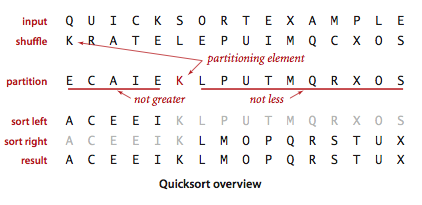The crux of the method is the partitioning process, which rearranges the array to make the following three conditions hold:

• The entry a[j] is in its final place in the array, for some j.

• No entry in a[lo] through a[j-1] is greater than a[j].

• No entry in a[j+1] through a[hi] is less than a[j].
We achieve a complete sort by partitioning, then recursively applying the method to the subarrays. It is a randomized algorithm, because it randomly shuffles the array before sorting it.

### Partitioning.

To complete the implementation, we need to implement the partitioning method. We use the following general strategy: First, we arbitrarily choose a[lo] to be the partitioning item—the one that will go into its final position. Next, we scan from the left end of the array until we find an entry that is greater than (or equal to) the partitioning item, and we scan from the right end of the array until we find an entry less than (or equal to) the partitioning item.The two items that stopped the scans are out of place in the final partitioned array, so we exchange them. When the scan indices cross, all that we need to do to complete the partitioning process is to exchange the partitioning item a[lo] with the rightmost entry of the left subarray (a[j]) and return its index j.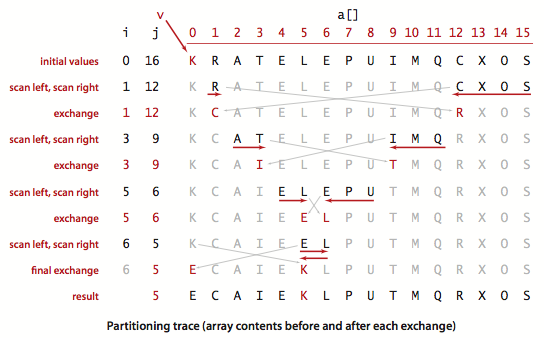### Quicksort.

Quick.java is an implementation of quicksort, using the partitioning method described above.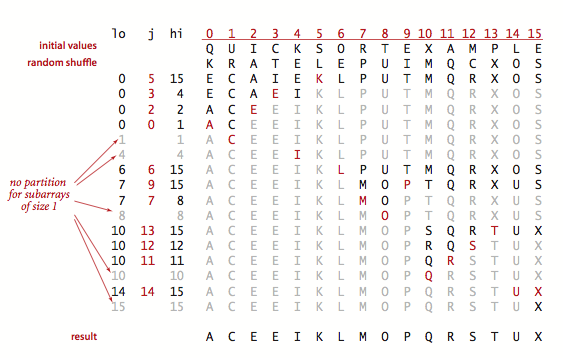### Implementation details.

There are several subtle issues with respect to implementing quicksort that are reflected in this code and worthy of mention.
• Partitioning inplace. If we use an extra array, partitioning is easy to implement, but not so much easier that it is worth the extra cost of copying the partitioned version back into the original.
• Staying in bounds. If the smallest item or the largest item in the array is the partitioning item, we have to take care that the pointers do not run off the left or right ends of the array, respectively.
• Preserving randomness. The random shuffle puts the array in random order. Since it treats all items in the subarrays uniformly, Quick.java has the property that its two subarrays are also in random order. This fact is crucial to the algorithm's predictability. An alternate way to preserve randomness is to choose a random item for partitioning within partition().
• Terminating the loop. Properly testing whether the pointers have crossed is a bit trickier than it might seem at first glance. A common error is to fail to take into account that the array might contain other keys with the same value as the partitioning item.
• Handling items with keys equal to the partitioning item's key. It is best to stop the left scan for items with keys greater than or equal to the partitioning item's key and the right scan for items less than or equal to the partitioning item's key. Even though this policy might seem to create unnecessary exchanges involving items with keys equal to the partitioning item's key, it is crucial to avoiding quadratic running time in certain typical applications.
• Terminating the recursion. A common mistake in implementing quicksort involves not ensuring that one item is always put into position, then falling into an infinite recursive loop when the partitioning item happens to be the largest or smallest item in the array.

### Proposition.

Quicksort uses ~2 N ln N compares (and one-sixth that many exchanges) on the average to sort an array of length N with distinct keys.

### Proposition.

Quicksort uses ~N2/2 compares in the worst case, but random shuffling protects against this case.

The standard deviation of the running time is about .65 N, so the running time tends to the average as N grows and is unlikely to be far from the average. The probability that quicksort will use a quadratic number of compares when sorting a large array on your computer is much less than the probability that your computer will be struck by lightning!

### Improvements.

Quicksort was invented in 1960 by C. A. R. Hoare, and it has been studied and refined by many people since that time.
• Cutoff to insertion sort. As with mergesort, it pays to switch to insertion sort for tiny arrays. The optimum value of the cutoff is system-dependent, but any value between 5 and 15 is likely to work well in most situations.
• Median-of-three partitioning. A second easy way to improve the performance of quicksort is to use the median of a small sample of items taken from the array as the partitioning item. Doing so will give a slightly better partition, but at the cost of computing the median. It turns out that most of the available improvement comes from choosing a sample of size 3 (and then partitioning on the middle item).

## Visualization.

QuickBars.java visualizes quicksort with median-of-3 partitioning and cutoff for small subarrays.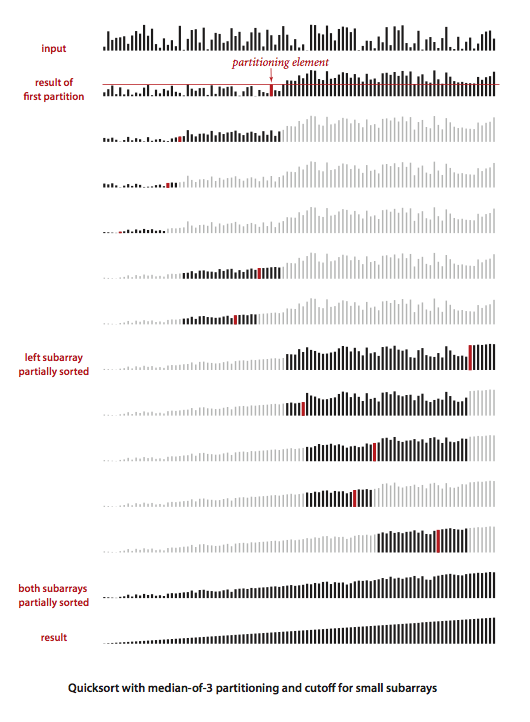## Entropy-optimal sorting.

Arrays with large numbers of duplicate sort keys arise frequently in applications. In such applications, there is potential to reduce the time of the sort from linearithmic to linear.

One straightforward idea is to partition the array into three parts, one each for items with keys smaller than, equal to, and larger than the partitioning item's key. Accomplishing this partitioning was a classical programming exercise popularized by E. W. Dijkstra as the Dutch National Flag problem, because it is like sorting an array with three possible key values, which might correspond to the three colors on the flag.

Dijkstra's solution is based on a single left-to-right pass through the array that maintains a pointer lt such that a[lo..lt-1] is less than v, a pointer gt such that a[gt+1..hi] is greater than v, and a pointer i such that a[lt..i-1] are equal to v, and a[i..gt] are not yet examined.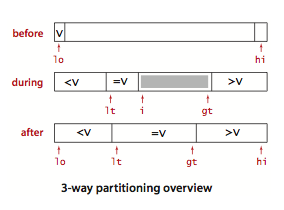Starting with i equal to lo we process a[i] using the 3-way compare given us by the Comparable interface to handle the three possible cases:

• a[i] less than v: exchange a[lt] with a[i] and increment both lt and i

• a[i] greater than v: exchange a[i] with a[gt] and decrement gt

• a[i] equal to v: increment i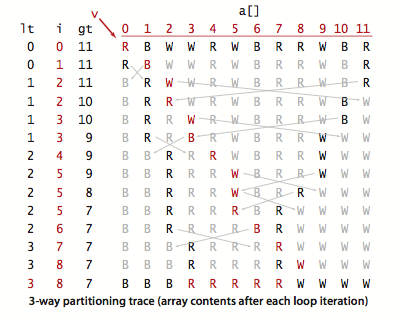Quick3way.java is an implementation of this method.

### Proposition.

Quicksort with 3-way partitioning is entropy-optimal.

## Visualization.

Quick3wayBars.java visualizes quicksort with 3-way partitioning.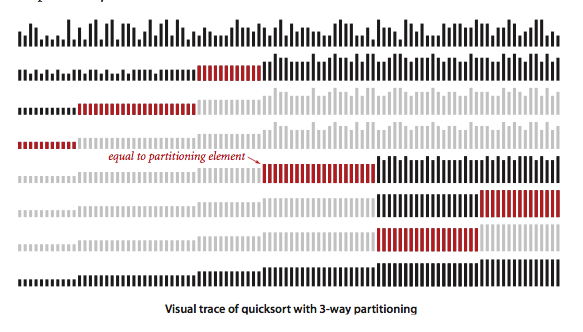#### Exercises

1. Show, in the style of the trace given with partition(), how that method partitions the array E A S Y Q U E S T I O N.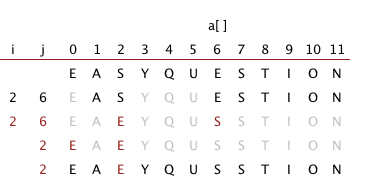2. Show, in the style of the quicksort trace, how quicksort sorts the array E A S Y Q U E S T I O N. (For the purposes of this exercise, ignore the initial shuffle.)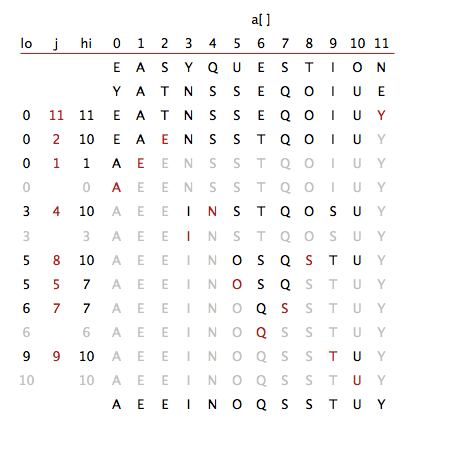3. Write a program Sort2distinct.java that sorts an array that is known to contain just two distinct key values.

4. About how many compares will Quick.sort() make when sorting an array of N items that are all equal?

Solution. ~ N lg N compares. Each partition will divide the array in half, plus or minus one.

5. Show, in the style of the trace given with the code, how the entropy-optimal sort first partitions the array B A B A B A B A C A D A B R A.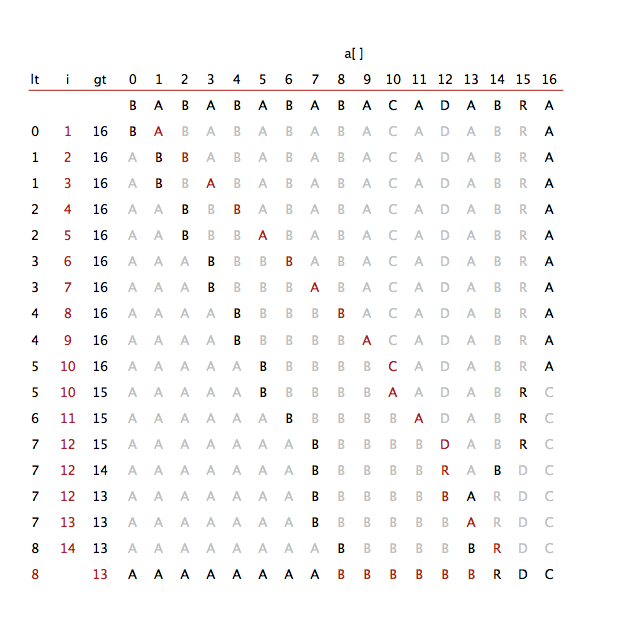#### Creative Problems

1. Nuts and bolts. (G. J. E. Rawlins). You have a mixed pile of N nuts and N bolts and need to quickly find the corresponding pairs of nuts and bolts. Each nut matches exactly one bolt, and each bolt matches exactly one nut. By fitting a nut and bolt together, you can see which is bigger. But it is not possible to directly compare two nuts or two bolts. Given an efficient method for solving the problem.

Hint: customize quicksort to the problem. Side note: only a very complicated deterministic O(N log N) algorithm is known for this problem.

2. Best case. Write a program QuickBest.java that produces a best-case array (with no duplicates) for Quick.sort(): an array of N distinct keys with the property that every partition will produce subarrays that differ in size by at most 1 (the same subarray sizes that would happen for an array of N equal keys). For the purposes of this exercise, ignore the initial shuffle.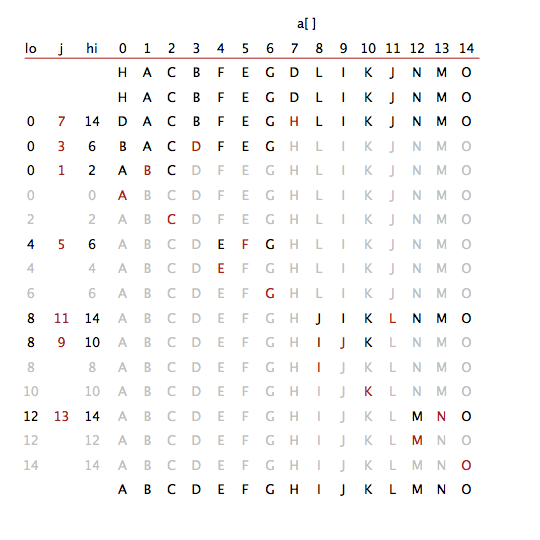3. Fast three-way partitioning. (J. Bentley and D. McIlroy). Implement an entropy-optimal sort QuickBentleyMcIlroy.java based on keeping equal keys at both the left and right ends of the subarray. Maintain indices p and q such that a[lo..p-1] that a[q+1..hi] are all equal to a[lo], an index i such that a[p..i-1] are all less than a[lo] and an index j such that a[j+1..q] are all greater than a[lo]. Add to the inner partitioning loop code to swap a[i] with a[p] (and increment p) if it is equal to v and to swap a[j] with a[q] (and decrement q) if it is equal to v before the usual comparisons of a[i] and a[j] with v.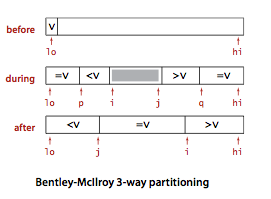After the partitioning loop has terminated, add code to swap the equal keys into position.

#### Web Exercises

1. QuickKR.java is one of the simplest quicksort implementations, and appears in K+R. Convince yourself that it is correct. How will it perform? All equal keys?
2. Randomized quicksort. Modify partition() so that it always chooses the partitioning item uniformly at random from the array (instead of shuffling the array initially). Compare the performance against Quick.java.
3. Antiquicksort. The algorithm for sorting primitive types in Java 6 is a variant of 3-way quicksort developed by Bentley and McIlroy. It is extremely efficient for most inputs that arise in practice, including inputs that are already sorted. However, using a clever technique described by M. D. McIlroy in A Killer Adversary for Quicksort, it is possible to construct pathological inputs that make the system sort run in quadratic time. Even worse, it overflows the function call stack. To see the sorting library in Java 6 break, here are some killer inputs of varying sizes: 10,000, 20,000, 50,000, 100,000, 250,000, 500,000, and 1,000,000. You can test them out using the program IntegerSort.java which takes a command line input N, reads in N integers from standard input, and sorts them using the system sort.
4. Bad partitioning. How does not stopping on equal keys make quicksort go quadratic when all keys are equal?

Solution. Here is the result of partitioning AAAAAAAAAAAAAAA when we don't stop on equal keys. It unevenly partitions the array into one subproblem of size 0 and one of size 14.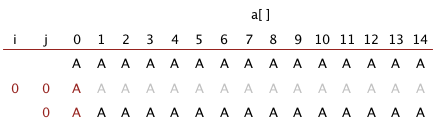Here is the result of partitioning AAAAAAAAAAAAAAA when we do stop on equal keys. It evenly partitions the array into two subproblems of size 7.5. Comparing an item against itself. Show that our implementation of quicksort can compare an item against itself, i.e., calls less(i, i) for some index i. Modify our implementation so that it never compares an item against itself.
6. Hoare's original quicksort. Implement a version of Hoare's original quicksort algorithm. It's similar to our two-way partitioning algorithm except that the pivot is not swapped into its final position. Instead, the pivot is left in one of the two subarrays, no element is fixed in its final position, and the two subarrays where the pointers cross are sorted recursively.

Solution. HoareQuick.java. We note that, while this verison is quite elegant, it does not preserve randomness in the subarrays. According to Sedgewick's PhD thesis, "this bias not only makes analysis of the method virtually impossible, it also slows down the sorting process considerably."

7. Dual-pivot quicksort. Implement a version of Yaroslavskiy's dual-pivot quicksort.

Solution. QuickDualPivot.java is an implementation that is very similar to Quick3way.java.

8. Three-pivot quicksort. Implement a version of three-pivot quicksort ala Kushagra-Ortiz-Qiao-Munro.
9. Number of compares. Give a family of arrays of length n for which the standard quicksort partitioning algorithm makes (i) n + 1 compares, (ii) n compares, (iii) n - 1 compares, or argue that no such family of arrays exist.

Solution: ascending order; descending order; none.